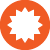#Structural Equation Modelling (SEM)

Course content
• Structural Equation Modeling: An Introduction
• Confirmatory Factor Analysis
• Testing a Structural Model
• Formative  constructs, higher-order CFA, and multi-group models
• Testing for mediation and moderation using SEM procedures and assessing measurement bias
• Reflective activities – use SEM procedures to address contemporary research problems
Learning Objectives
By the end of this course participants will be able to:
• Understand the distinguishing characteristics of Structural Equation Modeling
• Explain the type of relationships involved in SEM
• Understand the role of theory in developing structural equation models
• Understand the difference between a measurement model and a structural model
• Understand the differences between formative and reflective constructs
• Know how to represent a measurement and a structural model using a path diagram
• Test confirmatory factor analysis (CFA) and structural models
• Assess the goodness-of-fit of measurement and structural models
• Assess construct validity and reliability using SEM procedures
• Test structural models that involve mediating and moderating effects
• Perform multi-group analysis

Who is the program designed for:
Participants should be familiar with basic statistics and should know how to run a correlation analysis, linear regression and t-test. No prior knowledge of Structural Equation Modelling software is necessary.  The course is appropriate for doctoral students and academic researchers that use quantitative research methodologies.